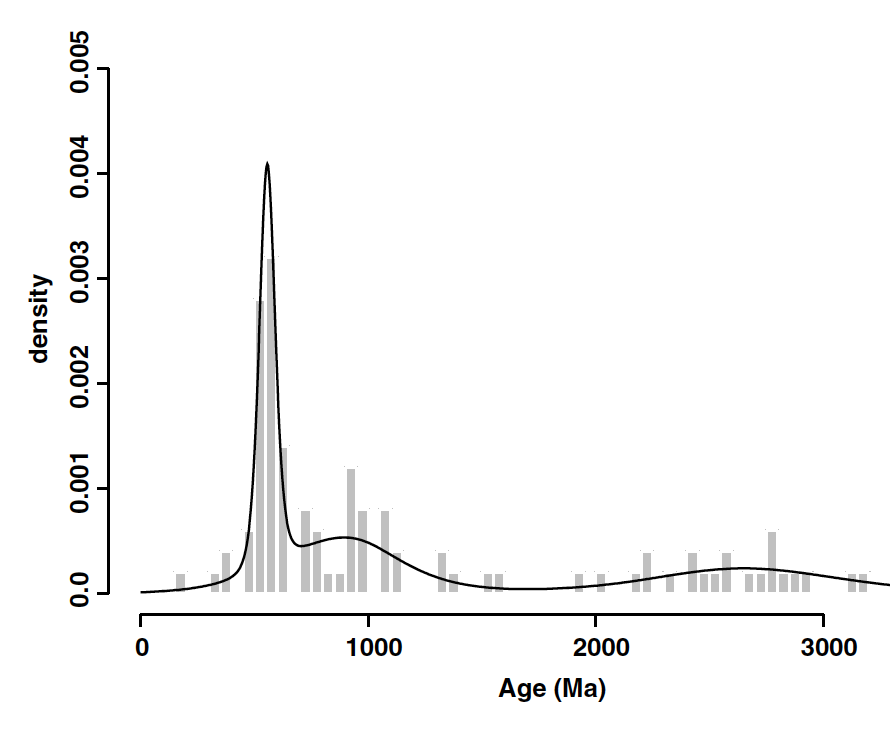## BayesMixQt

### Overview

The BayesMixQt application allows us to infer the probability distribution of the number of component distributions from a distribution of input ages (+ associated errors), under the assumption of either Normal (Gaussian) or Skew-t distributions. Furthermore, we infer the parameters defined each component distribution (such as the mean, standard deviation, left and right skewness), and also the proportion of each individual component distribution contributing to the total distribution. Each parameter has an uncertainty and these are characterized through the 95% credible range.

The approach implemented uses Reversible Jump Markov chain Monte Carlo (RJ-MCMC), which is a iterative sampling strategy, allowing for the dimension of the model parameter space to change (i.e. the number of component distributions and the parameters associated with each component distribution). We use a Bayesian formulation, which requires the specification of both prior distributions and proposal distributions. For the prior distributions, these are generally set to default values, so the user does not need to specify the form. For the proposal distributions, default values can be used, and adjusted according to the acceptance rate of proposed parameter values. The acceptance rates are monitored during a run and output at the end, allowing the user to update their values, interactively.

The results can be examined through a series of plots, and summary statistics written to the screen (and, optionally to an output file).Figure 1. Sample result.

A user guide and program binaries are available:

User Guide to BayesMix.pdf 670KB
Mac 21MB
Windows 16M

Alternatively, it can be downloaded from the author's web site using these instructions:

Accessing QTQt and BayesMixQT for Windows or Macintosh.pdf 670KB.

### References

The main references for the methodology used in this application is:

Bayesian Mixture Modelling in Geochronology via Markov Chain Monte Carlo, Jarsa, A., Stephens, D., Gallagher, K. and Holmes, C., Mathematical Geology, doi:10.1007/s11004-005-9019-3, 2005.

Markov chain Monte Carlo (MCMC) sampling methods to determine optimal models, model resolution and model choice for Earth Science problems, Gallagher, K., Charvin, K., Nielsen, S., Sambridge, M. and Stephenson, J., Marine and Petroleum Geology, doi:10.1016/j.marpetgeo.2009.01.003, 2009

Additional references describing the use of RJ-MCMC methods.